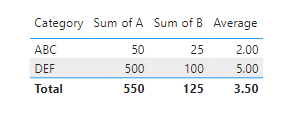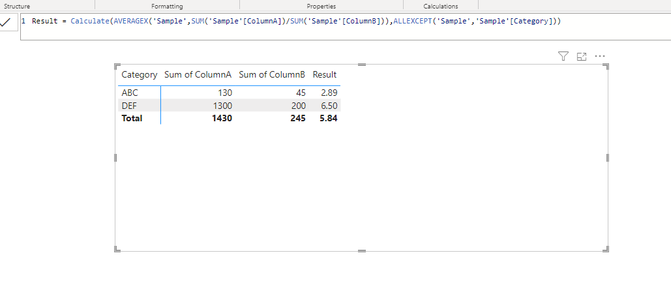cancel
Showing results for
Did you mean:Helper I

## Show average of column values in grand total row

Hi,

I will try to explain my issue by taking an example. Please refer below screenshot. I need help to implement Yellow highlighted part.There is a column called "Result" which is a measure by dividing Column A and Column B.

In the Grand total row of the matrix, I want to show "Result" value as an Average.

So from the above screenshot value, I want value as 3.5. But instead I am getting 4.4 because 550/125.

How to achieve 3.5 as a value in "Result" grand total.

1 ACCEPTED SOLUTIONHelper I

Hi,

I figured out the solution in case any one needed this. This may not be optimized solution but one can optimize as per the requirement.

So I have created four measures basically.

``````MeasureA =SUM('Sample'[ColumnA])

MeasureB = SUM('Sample'[ColumnB])

IntermediateMeasure = MeasureA/MeasureB

ResultMeasure =

VAR TotalResults = SUM('Sample'[ColumnA]) / SUM('Sample'[ColumnB])
VAR DistinctCategoryCount = DISTINCTCOUNT('Sample'[Category])
RETURN
IF(
ISFILTERED('Sample'[Category]),
DIVIDE(TotalResults, DistinctCategoryCount),
AVERAGEX(VALUES('Sample'[Category]), [IntermediateMeasure ])
)``````

Here is the screenshot of the result :5 REPLIES 5Helper I

Hi,

I figured out the solution in case any one needed this. This may not be optimized solution but one can optimize as per the requirement.

So I have created four measures basically.

``````MeasureA =SUM('Sample'[ColumnA])

MeasureB = SUM('Sample'[ColumnB])

IntermediateMeasure = MeasureA/MeasureB

ResultMeasure =

VAR TotalResults = SUM('Sample'[ColumnA]) / SUM('Sample'[ColumnB])
VAR DistinctCategoryCount = DISTINCTCOUNT('Sample'[Category])
RETURN
IF(
ISFILTERED('Sample'[Category]),
DIVIDE(TotalResults, DistinctCategoryCount),
AVERAGEX(VALUES('Sample'[Category]), [IntermediateMeasure ])
)``````

Here is the screenshot of the result :Solution Sage

Hello @Kandarp ,

Use AVERAGEX instead of AVERAGE directly to get the overall average work the same.

``Average = AVERAGEX('Table','Table'[A]/'Table'[B])``

Output looks like this:If this post helps, then please consider accepting it as the solution to help other members find it more quickly. Thank You!!Helper I

@Kishore_KVN Thanks this is perfect solution. But It will not work if we have multiple row with same category. In my case I have mutliple rows with same category name.

For example, if we have below data :Then suggested solution will not work. Expected result is 4.695 but this formula shows 5.84. See below :If you have any solution on this, please suggest. Thanks in advance.Solution Sage

Hello @Kandarp  in that case you have to add grouping for the category.

``Average = Calculate(AVERAGEX('Table',sum('Table'[A])/sum('Table'[B])),ALLEXCEPT(Table,'Table'[Category]))``

If this post helps, then please consider accepting it as the solution to help other members find it more quickly. Thank You!!Helper I

@Kishore_KVN Unfortunately it is giving the same result :